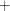### TEST Statement

`TEST` <name> test1 < , test2 …> < ,/ options > ;

The TEST statement performs tests of linear hypotheses on the model parameters.

The TEST statement applies only to parameters estimated in the model. You can specify any number of TEST statements.

Each test is written as an expression optionally followed by an equal sign (=) and a second expression:

`expression <= expression> `

Test expressions can be composed of variable names, multiplication (), addition (), and subtraction () operators, and constants. Variables named in test expressions must be among the variables estimated by the model.

If you specify only one expression in a TEST statement, that expression is tested against zero. For example, the following two TEST statements are equivalent:

```   test a + b;

test a + b = 0;
```

When you specify multiple tests on the same TEST statement, a joint test is performed. For example, the following TEST statement tests the joint hypothesis that both of the coefficients on `a` and `b` are equal to zero:

```   test a, b;
```

To perform separate tests rather than a joint test, use separate TEST statements. For example, the following TEST statements test the two separate hypotheses that `a` is equal to zero and that `b` is equal to zero:

```   test a;
test b;
```

You can use the following options in the TEST statement:

WALD

specifies that a Wald test be computed. WALD is the default.

LM

RAO
LAGRANGE

specifies that a Lagrange multiplier test be computed.

LR
LIKE

specifies that a pseudo-likelihood ratio test be computed.

ALL

requests all three types of tests.

OUT=

specifies the name of an output SAS data set that contains the test results. The format of the OUT= data set produced by the TEST statement is similar to that of the OUTEST= data set.